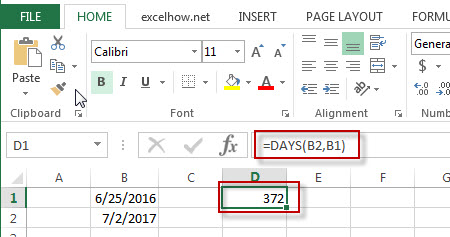# Excel Days Function

This post will guide you how to use Excel DAYS function with syntax and examples in Microsoft excel.

### Description

The Excel DAYS function returns the number of days between two dates.

The DAYS function is a build-in function in Microsoft Excel and it is categorized as a DATE and TIME Function.

The DAYS function is available in Excel 2016, Excel 2013.

### Syntax

The syntax of the DAYS function is as below:

= DAYS (end_date,start_date)

### Example

The below examples will show you how to use Excel DAYS Function to return the number of days between end_date and start_date.

#1 =DAYS (B2,B1)Note: the above excel formula retruns the number of days (372) between the end date (7/2/2017) and start date (6/25/2016).